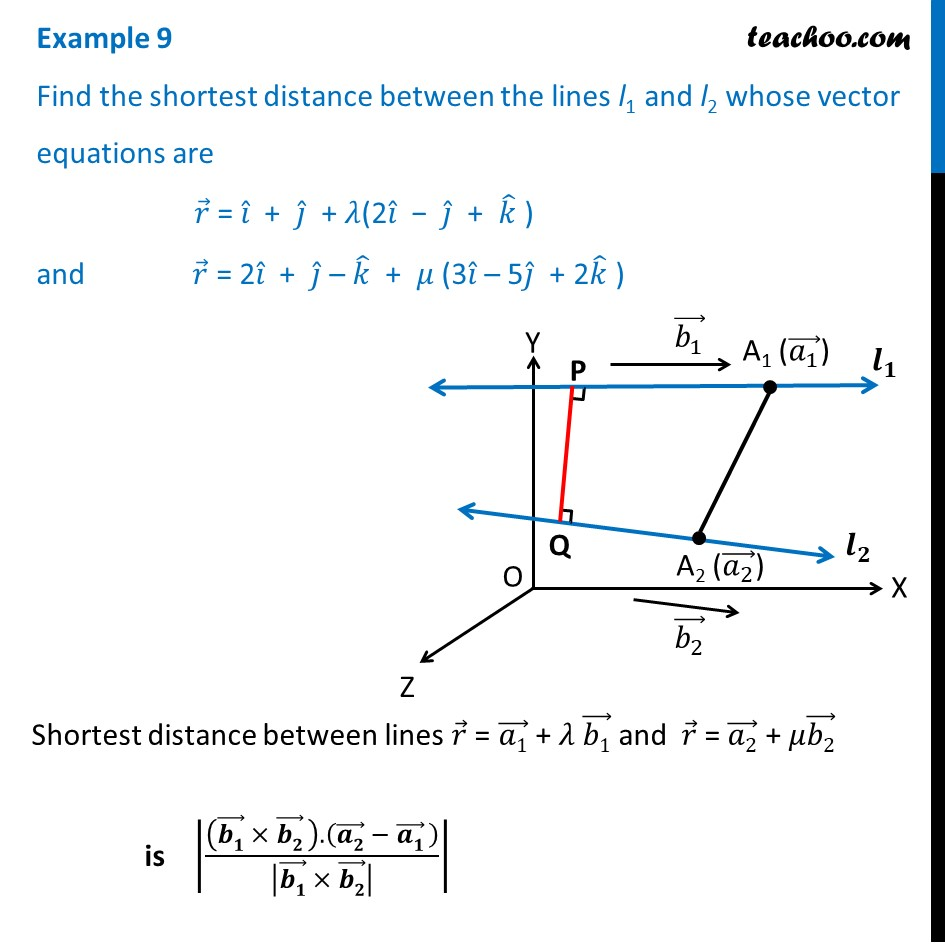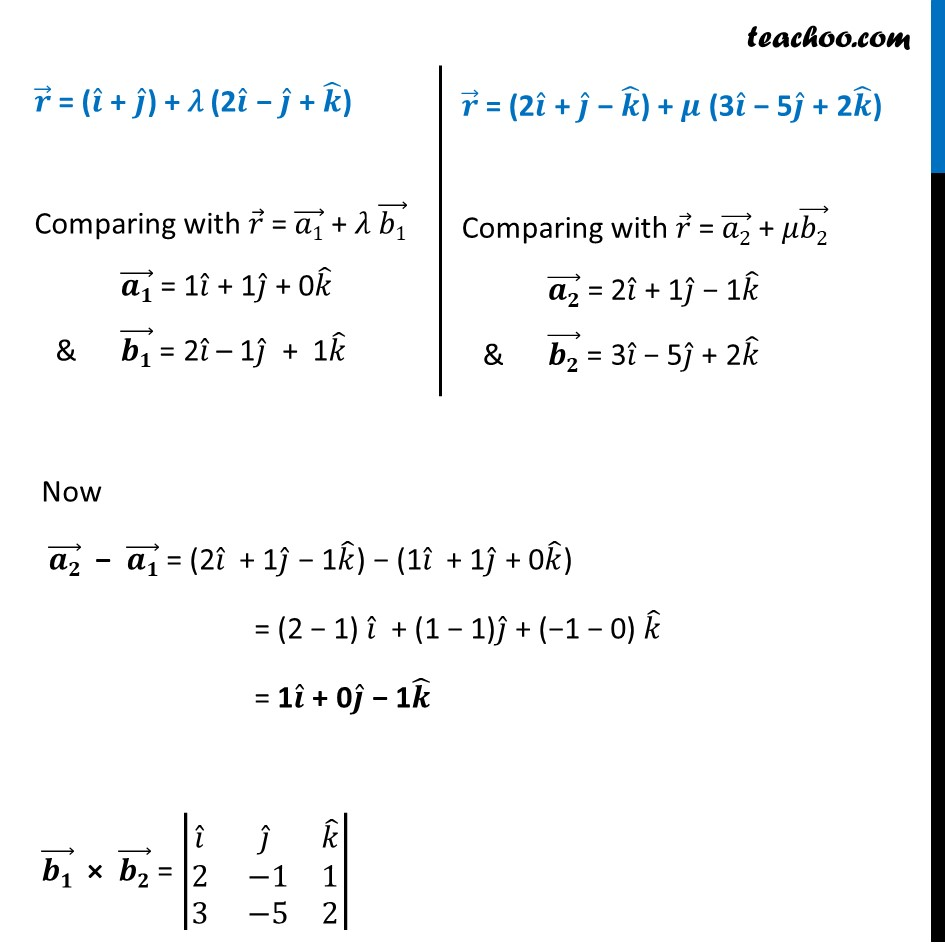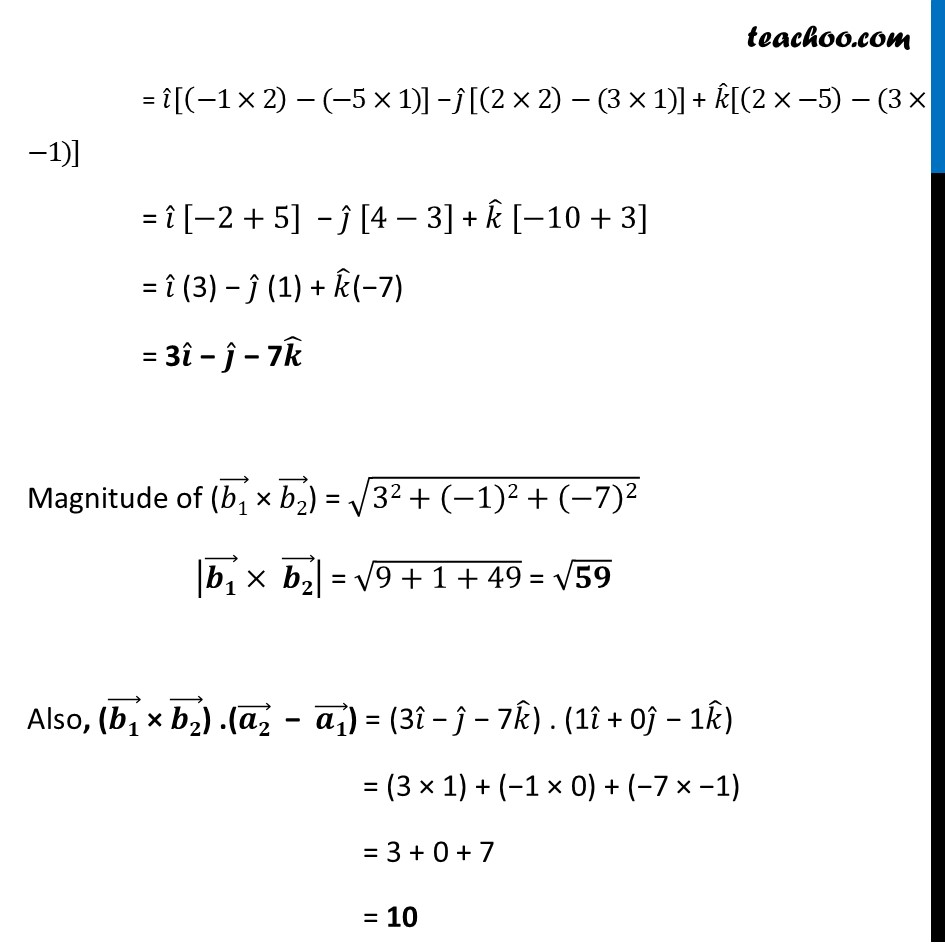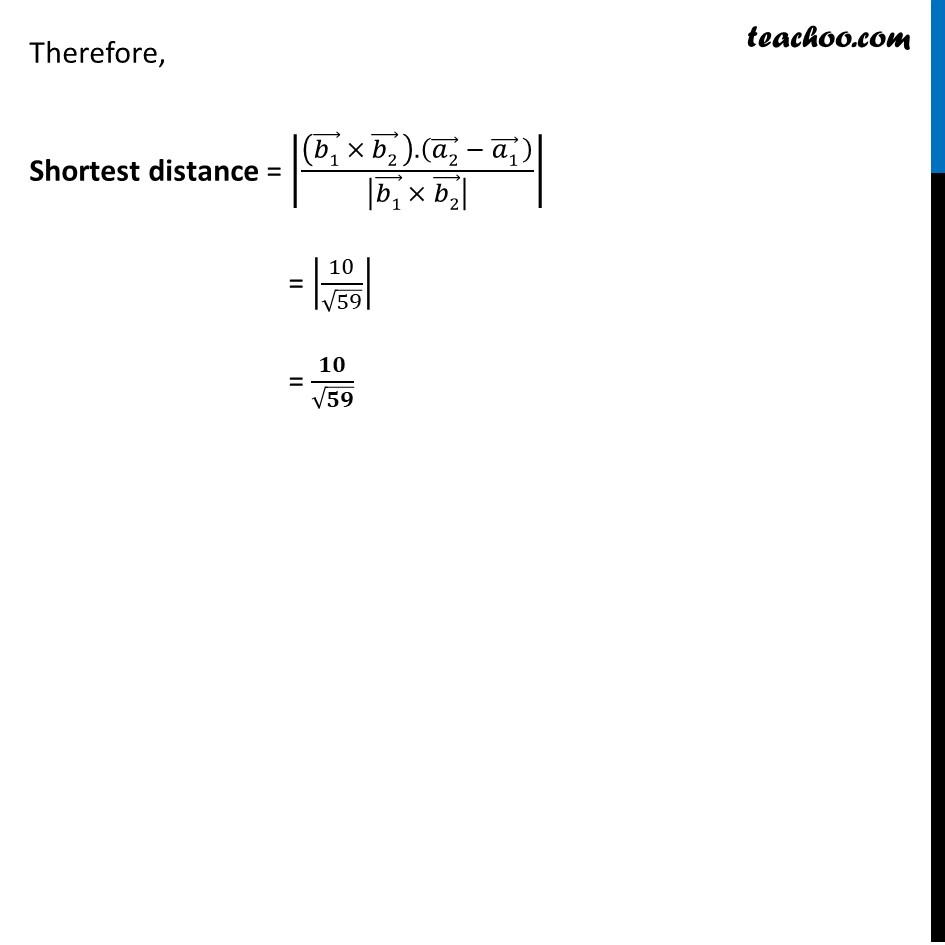Examples

Chapter 11 Class 12 Three Dimensional Geometry
Serial order wiseLearn in your speed, with individual attention - Teachoo Maths 1-on-1 Class

### Transcript

Example 9 Find the shortest distance between the lines l1 and l2 whose vector equations are 𝑟 ⃗ = 𝑖 ̂ + 𝑗 ̂ + 𝜆(2𝑖 ̂ − 𝑗 ̂ + 𝑘 ̂ ) and 𝑟 ⃗ = 2𝑖 ̂ + 𝑗 ̂ – 𝑘 ̂ + 𝜇 (3𝑖 ̂ – 5𝑗 ̂ + 2𝑘 ̂ )Shortest distance between lines 𝑟 ⃗ = (𝑎1) ⃗ + 𝜆 (𝑏1) ⃗ and 𝑟 ⃗ = (𝑎2) ⃗ + 𝜇(𝑏2) ⃗ is |(((𝒃𝟏) ⃗ × (𝒃𝟐) ⃗ ).((𝒂𝟐) ⃗ − (𝒂𝟏) ⃗ ))/|(𝒃𝟏) ⃗ × (𝒃𝟐) ⃗ | | 𝒓 ⃗ = (𝒊 ̂ + 𝒋 ̂) + 𝜆 (2𝒊 ̂ − 𝒋 ̂ + 𝒌 ̂) Comparing with 𝑟 ⃗ = (𝑎1) ⃗ + 𝜆 (𝑏1) ⃗ (𝒂𝟏) ⃗ = 1𝑖 ̂ + 1𝑗 ̂ + 0𝑘 ̂ & (𝒃𝟏) ⃗ = 2𝑖 ̂ – 1𝑗 ̂ + 1𝑘 ̂ 𝒓 ⃗ = (2𝒊 ̂ + 𝒋 ̂ − 𝒌 ̂) + 𝝁 (3𝒊 ̂ − 5𝒋 ̂ + 2𝒌 ̂) Comparing with 𝑟 ⃗ = (𝑎2) ⃗ + 𝜇(𝑏2) ⃗ (𝒂𝟐) ⃗ = 2𝑖 ̂ + 1𝑗 ̂ − 1𝑘 ̂ & (𝒃𝟐) ⃗ = 3𝑖 ̂ − 5𝑗 ̂ + 2𝑘 ̂ Now (𝒂𝟐) ⃗ − (𝒂𝟏) ⃗ = (2𝑖 ̂ + 1𝑗 ̂ − 1𝑘 ̂) − (1𝑖 ̂ + 1𝑗 ̂ + 0𝑘 ̂) = (2 − 1) 𝑖 ̂ + (1 − 1)𝑗 ̂ + (−1 − 0) 𝑘 ̂ = 1𝒊 ̂ + 0𝒋 ̂ − 1𝒌 ̂ (𝒃𝟏) ⃗ × (𝒃𝟐) ⃗ = |■8(𝑖 ̂&𝑗 ̂&𝑘 ̂@2& −1&1@3& −5&2)| = 𝑖 ̂ [(−1×2)−(−5×1)] − 𝑗 ̂ [(2×2)−(3×1)] + 𝑘 ̂[(2×−5)−(3×−1)] = 𝑖 ̂ [−2+5] − 𝑗 ̂ [4−3] + 𝑘 ̂ [−10+3] = 𝑖 ̂ (3) − 𝑗 ̂ (1) + 𝑘 ̂(−7) = 3𝒊 ̂ − 𝒋 ̂ − 7𝒌 ̂ Magnitude of ((𝑏1) ⃗ × (𝑏2) ⃗) = √(32+(−1)2+(−7)^2 ) |(𝒃𝟏) ⃗× (𝒃𝟐) ⃗ | = √(9+1+49) = √𝟓𝟗 Also, ((𝒃𝟏) ⃗ × (𝒃𝟐) ⃗) .((𝒂𝟐) ⃗ − (𝒂𝟏) ⃗) = (3𝑖 ̂ − 𝑗 ̂ − 7𝑘 ̂) . (1𝑖 ̂ + 0𝑗 ̂ − 1𝑘 ̂) = (3 × 1) + (−1 × 0) + (−7 × −1) = 3 + 0 + 7 = 10 Therefore, Shortest distance = |(((𝑏1) ⃗ × (𝑏2) ⃗ ).((𝑎2) ⃗ − (𝑎1) ⃗ ))/|(𝑏1) ⃗ × (𝑏2) ⃗ | | = |10/√59| = 𝟏𝟎/√𝟓𝟗